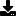Don't have an account yet? Register

# 3D Mathematical Plot

by kmmatney (4 Submissions)
Category: Complete Applications
Compatability: Visual Basic 3.0
Difficulty: Unknown Difficulty
Date Added: Wed 3rd February 2021
Rating:(5 Votes)

This project shows how to take any custom equation that is a function of X and Y, and plot it in a picture box. The plot is a rudimentary 2D colored contour plot, but it does look pretty. This project has several useful components:
1) An equation parser class with built-in error checking
2) Color mixing algorithms with a customized interface
3) Shows how to plot XYZ values in a picture box
Cool stuff...

Assumes

Rate 3D Mathematical PlotDownload 3D Mathematical Plot (16 KB)